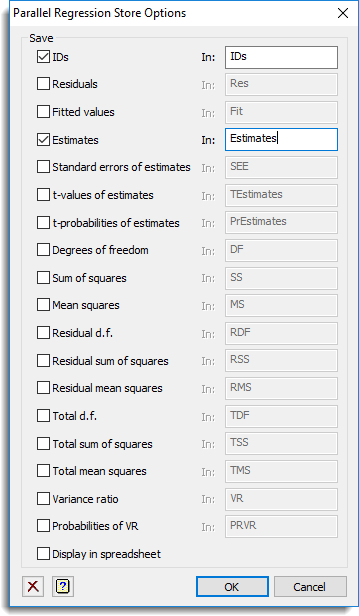1. Home
2. Parallel Regression Store Options

# Parallel Regression Store Options

This dialog lets you save results from a Parallel regression. These results are saved when an analysis is run and must be specified before running an analysis.

1. After selecting the appropriate boxes, type names for the data structures into the corresponding In: fields.## Save

The table below indicates the type of structures formed for each item.

 IDs Text The list of Unit identifiers to index the results. Residuals Matrix The residuals from the fitted regression model will be saved in a matrix of this name. The units in the model will be in the columns of the matrix and there will be one row for each probe. Fitted values Matrix The fitted values for the fitted regression model will be saved in a matrix of this name. The units in the model will be in the columns of the matrix and there will be one row for each probe. Estimates Matrix The estimates for each model term will be saved in a matrix of this name. The model terms will be in the columns of the matrix and there will be one row for each probe. Standard errors of estimates Matrix The standard errors of the estimates for each model term will be saved in a matrix of this name. The model terms will be in the columns of the matrix and there will be one row for each probe. T-values of estimates Matrix The T-values (i.e. the estimate divided by its standard error) of the estimates for each model term will be saved in a matrix of this name. The model terms will be in the columns of the matrix and there will be one row for each probe. T-probabilities of estimates Matrix The probability of the T-values of the estimates for each model term will be saved in a matrix of this name. The model terms will be in the columns of the matrix and there will be one row for each probe. Degrees of freedom Pointer The degrees of freedom for each treatment will be saved in unnamed variates pointed to by this pointer. These are stored in unnamed variates for each treatment term which are pointed to by this pointer. Sums of squares Pointer The sums of squares for each treatment will be saved in unnamed variates pointed to by this pointer. These are stored in unnamed variates for each treatment term which are pointed to by this pointer. Mean squares Pointer The mean of squares (i.e. the sums of squares divided by its degrees of freedom) for each model term will be saved in unnamed variates pointed to by this pointer. Residual DF Variate The residual degrees of freedom of the regression model fitted. Residual sum of squares Variate The residual sums of squares of the regression model fitted. Residual mean squares Variate The residual mean squares (i.e. the sums of squares divided by its degrees of freedom) of the regression model fitted. Total DF Variate The total degrees of freedom will be saved in a variate of this name. Total sum of squares Variate The total sum of squares will be saved in a variate of this name. Total mean squares Variate The total mean of squares (i.e. the total sum of squares divided by the total degrees of freedom) will be saved in a variate of this name. Variance ratio Pointer The variance or F ratio (i.e. the model term mean squares divided by the residual mean squares) for each model term will be saved in unnamed variates pointed to by this pointer. Probability of VR Pointer The probability of a variance ratio (i.e. F statistic) being more extreme than the observed the F ratio for each model term will be saved in unnamed variates pointed to by this pointer.Clear Clear all fields and list boxes.Help Open the Help topic for this dialog.